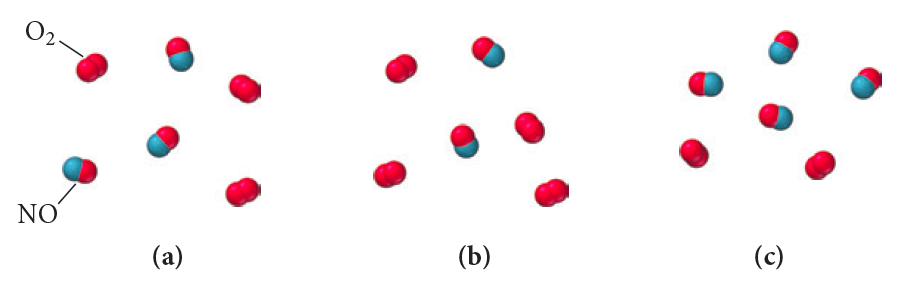# Problem: This reaction was experimentally determined to be first order with respect to O2 and second order with respect to NO: O2(g) +2 NO(g) → 2 NO2(g)The diagrams shown here represent reaction mixtures in which the number of each type of molecule represents its relative initial concentration. Which mixture has the fastest initial rate?

###### FREE Expert Solution

Rate Law can be assumed as:

83% (498 ratings)###### Problem Details

This reaction was experimentally determined to be first order with respect to O2 and second order with respect to NO: O2(g) +2 NO(g) → 2 NO2(g)

The diagrams shown here represent reaction mixtures in which the number of each type of molecule represents its relative initial concentration. Which mixture has the fastest initial rate?Frequently Asked Questions

What scientific concept do you need to know in order to solve this problem?

Our tutors have indicated that to solve this problem you will need to apply the Rate Law concept. You can view video lessons to learn Rate Law. Or if you need more Rate Law practice, you can also practice Rate Law practice problems.

What professor is this problem relevant for?

Based on our data, we think this problem is relevant for Professor Mccurdy's class at UCD.